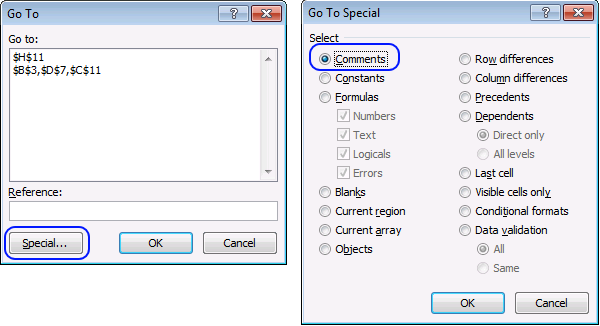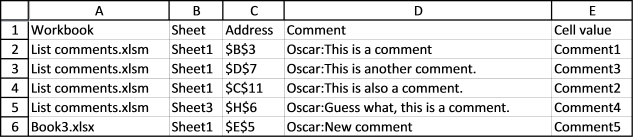Author: Oscar Cronquist Article last updated on January 19, 2018

Did you know that you can select all comments in the current sheet? Press F5, click "Special..." button, select "Comments" and then click OK.What happens if we record a macro while performing these actions? This is what the macro recorder returns:

```Sub Macro1()
End Sub
```

We can use this code to retrieve all comments, their cell values, addresses and so on.

### List all comments in a sheet

The following code iterates through each cell containing a comment and saves the cell value, comment and address to a new sheet.

```Sub Macro1()
Dim WS As Worksheet
Dim Rng As Range
Dim cell As Variant
Dim i As Single

WS.Range("B1") = "Comment"
WS.Range("C1") = "Cell value"
i = 2
For Each cell In Rng
WS.Range("B" & i) = cell.Comment.Text
WS.Range("C" & i) = cell.Value
i = i + 1
Next cell
End Sub
```

### Build a list of comments from all sheets in a workbook to a new sheet

This code checks all sheets in the current workbook for comments.

```Sub Macro2()
Dim WS As Worksheet, WoSh As Worksheet
Dim Rng As Range
Dim cell As Variant
Dim i As Single

WS.Range("A1") = "Sheet"
WS.Range("C1") = "Comment"
WS.Range("D1") = "Cell value"
i = 2
For Each WoSh In ActiveWorkbook.Worksheets
On Error Resume Next
If Not Rng Is Nothing Then
For Each cell In Rng
WS.Range("A" & i) = WoSh.Name
WS.Range("C" & i) = cell.Comment.Text
WS.Range("C" & i).WrapText = False
WS.Range("D" & i) = cell.Value
i = i + 1
Next cell
End If
Set Rng = Nothing
Next WoSh

End Sub
```

### Build a list of comments from all sheets in all open workbooks

This code checks all open workbooks for comments.

```Sub Macro3()
Dim WS As Worksheet, WoSh As Worksheet
Dim WB As Workbook
Dim Rng As Range
Dim cell As Variant
Dim i As Single

WS.Range("A1") = "Workbook"
WS.Range("B1") = "Sheet"
WS.Range("D1") = "Comment"
WS.Range("E1") = "Cell value"
i = 2
For Each WB In Workbooks
For Each WoSh In WB.Worksheets
On Error Resume Next
If Not Rng Is Nothing Then
For Each cell In Rng
WS.Range("A" & i) = WB.Name
WS.Range("B" & i) = WoSh.Name
WS.Range("D" & i) = cell.Comment.Text
WS.Range("D" & i).WrapText = False
WS.Range("E" & i) = cell.Value
i = i + 1
Next cell
End If
Set Rng = Nothing
Next WoSh
Next WB
Columns("A:E").AutoFit
End Sub
```### Build a list of comments from all workbooks in a folder

I have reused some code from this macro: Search all workbooks in a folder

```Sub Macro4()
Dim WS As Worksheet
Dim myfolder As String
Dim Str As String
Dim a As Single
Dim sht As Worksheet
Dim c As Range

With Application.FileDialog(msoFileDialogFolderPicker)
.Show
myfolder = .SelectedItems(1) & "\"
End With
WS.Range("A1") = "Workbook"
WS.Range("B1") = "Sheet"
WS.Range("D1") = "Comment"
WS.Range("E1") = "Cell value"

a = 0
Value = Dir(myfolder)
Do Until Value = ""
If Value = "." Or Value = ".." Then
Else
If Right(Value, 3) = "xls" Or Right(Value, 4) = "xlsx" Or Right(Value, 4) = "xlsm" Then
On Error Resume Next
If Err.Number > 0 Then
WS.Range("A2").Offset(a, 0).Value = Value & " : Password protected"
a = a + 1
Else
On Error GoTo 0
For Each sht In ActiveWorkbook.Worksheets
On Error Resume Next
If Not c Is Nothing Then
For Each cell In c
WS.Range("A2").Offset(a, 0).Value = Value
WS.Range("B2").Offset(a, 0).Value = sht.Name
WS.Range("D2").Offset(a, 0).Value = cell.Comment.Text
WS.Range("D2").Offset(a, 0).WrapText = False
a = a + 1
Next cell
End If
Set c = Nothing
Next sht
End If
Workbooks(Value).Close False
On Error GoTo 0
End If
End If
Value = Dir
Loop
Cells.EntireColumn.AutoFit
End Sub
```

### Interesting articles you must read

List all open workbooks and corresponding sheets (vba)

Extract cell references from all cell ranges populated with values in a sheet